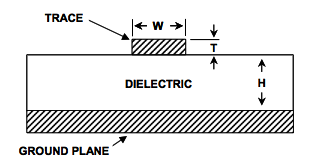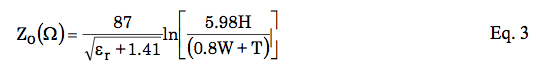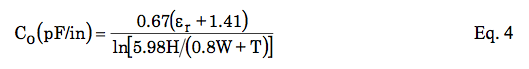news from the lab…
Designing 75Ohm traces
Categories: Synkie, video

Found this interesting application note “Microstrip and Stripline Design” http://www.analog.com/static/imported-files/tutorials/MT-094.pdf

with a formula to calculate PCB traces to have a 75 Ohm impedance:For a given PCB laminate and copper weight, note that all parameters will be predetermined except for W, the width of the signal trace. Eq. 3 can then be used to design a PCB trace to match the impedance required by the circuit. For the signal trace of width W and thickness T, separated by distance H from a ground (or power) plane by a PCB dielectric with dielectric constant εr, the characteristic impedance is:Note that in these expressions, measurements are in common dimensions (mils).
These transmission lines will have not only a characteristic impedance, but also capacitance. This can be calculated in terms of pF/in as shown in Eq. 4.As an example including these calculations, a 2-layer board might use 20-mil wide (W), 1 ounce (T=1.4) copper traces separated by 10-mil (H) FR-4 (εr = 4.0) dielectric material. The resulting impedance for this microstrip would be about 50 Ω. For other standard impedances, for example the 75-Ω video standard, adjust “W” to about 8.3 mils.

I’m using PCB prototyping material from Bungard with 1.5mm thickness and 35µm copper, FR4, so that translates to

• T = 1.377952755907 mil
• H = 59.0551181103 mil

Using above formula I see that I’d have to route 60.8mil (!) traces to achieve 75 Ohms impedance. Seems really fat to me. Am I right with my calculations or have I missed something ????

1 Comment to “Designing 75Ohm traces”

1. Arne says:

thats why hf engineers use material with higher dielectric constant.
Or you use multilayer PCBs where H should be much smaller.
Or you try to design a matching network.

Are you sure you need matched traces for 6.5 Mhz signals which got a wavelength in vacuum of 46m? (wavelength in microstrip is different but still large)